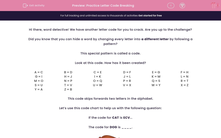# Practice Letter Code Breaking

In this worksheet, students will turn words into code and turn coded words back into their original words. This will develop student's encoding and problem solving skills.This content is premium and exclusive to EdPlace subscribers.Key stage:  KS 2

Curriculum topic:   Verbal Reasoning

Curriculum subtopic:   Letter Code Breaking

Difficulty level:#### Worksheet Overview

Hi there, word detective! We have another letter code for you to crack. Are you up to the challenge?

Did you know that you can hide a word by changing every letter into a different letter by following a pattern?

This special pattern is called a code.

Look at this code. How has it been created?

 A = C B = D C = E D = F E = G F = H G = I H = J I = K J = L K = M L = N M = O N = P O = Q P = R Q = S R = T S = U T = V U = W V = X W = Y X = Z Y = A Z = B

This code skips forwards two letters in the alphabet.

Let’s use this code chart to help us with the following question:

If the code for CAT is ECV...

The code for DOG is ____.If we find D, then O, then G in the code chart, we can work out that the code FQI represents the word DOG.

Sometimes, we have to work backwards from a given code. Let’s look at this question as an example:

If RGC is the code for PEA, then VQR is the code for ____.

We need to start on the right-hand side and find the letters V, then Q, then R and record the associated letters.  If we do this, we find that the word is TOP.Pssst!!             Here's a handy hint to help you reach superstar status:             You may need to keep a pencil and paper handy to do your working out on. Use the code chart to work out the code for each word.

In this activity, you will need to be a code cracker and work out which code represents each word. Remember that if you are working backwards from a code, you will need to look at which letters changed to make them.

Good luck, code cracker!

### What is EdPlace?

We're your National Curriculum aligned online education content provider helping each child succeed in English, maths and science from year 1 to GCSE. With an EdPlace account you’ll be able to track and measure progress, helping each child achieve their best. We build confidence and attainment by personalising each child’s learning at a level that suits them.

Get started